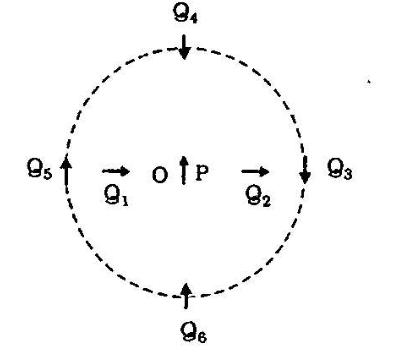Home/Class 12/Physics/

## QuestionPhysicsClass 12

Figure shows a small magnetised needle $$P$$  placed at a point $$O$$ . The arrow shows the direction of its magnetic moment. The other arrows show different positions $$($$ and orientations of the magnetic moment $$)$$ of another identical magnetised needle $$Q$$ . In which configuration the system is not in equilibrium?
Which configuration corresponds to the lowest potential energy among all the configurations shown?To solve this question, we need to know about the interaction of the magnetic moments with the magnetic fields.
Now, the potential energy of the configuration arises due to the potential energy of one dipole $$($$ say, $$Q$$  in the magnetic field due to other $$\left(P\right)$$ .We know that the field due to P is given by:
$$\overrightarrow B_p=\frac{-\mu _0}{4\pi }\frac{\overrightarrow m_p}{r^3}$$  $$($$ on the normal bisector $$)$$
$$\overrightarrow B_p=\frac{\mu _0}{4\pi }\frac{2\overrightarrow m_p}{r^3}$$            $$($$ on the axis $$)$$
Where, $$m_p$$ is the magnetic moment of the dipole $$\mathit{P.}$$
Equilibrium is stable when $$m_0$$  is parallel to $$B_P$$ , and unstable when it is anti-parallel to $$B_p$$  For instance for the conflguration $$g_3$$  for which $$g$$  is along the perpendicular bisector of the dipole $$P$$ , the magnetic moment of $$8$$  is. parallel to the magnetic field at the position $$3$$ . Hence $$Q_3$$  is stable. Thus,
Configuration corresponding to the lowest potential energy among all the configurations shown is $$PQ_1\text{ and }PQ_2$$